3.2 Sets- Part 1:

3.2 Example 1:

 In topic 1.1 we have studied different type of numbers and let us try to represent them using set notations.   If     N = {Set of natural numbers},     W={Set of whole Numbers},     Z= {Set of integers} and     Q= {set of rational numbers}     Then  N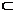WZQ     Note All these are infinite sets.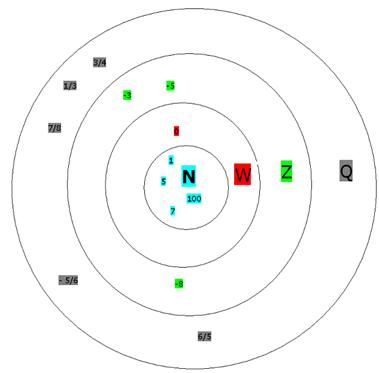3.2 Example 2: Let us consider the sets U = {Oxygen, Nitrogen, Hydrogen, Carbon dioxide, Sodium, Carbon, Calcium} A = {Oxygen, Nitrogen, Hydrogen}

B = {Sodium, Carbon, Calcium}

 Note that both A and B are subsets of U. Definition : 1) The ‘union’ (represented by the symbol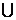) of two sets is defined as a set of all those elements which are either in A or in B or in both A and B. AB is pronounced as A union B. Thus in this example AB = {Oxygen, Nitrogen, Hydrogen, Sodium, Carbon, Calcium}. In the adjoining figure, the portion represented by grey colors is AB. 2. The ‘intersection ’(represented by the symbol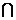)  of  two sets is defined as a set of all those elements which  are present  both in A and B..  The Symbolis pronounced as intersection. AB is pronounced as A intersection B In this example, note that sets A and B do not have any common element. When two sets do not have common elements then they are called  ‘disjoint’ sets. Note that in this example A and B are disjoint sets as they do not have any common element. Therefore AB ={ }=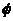(Null set) The representation of sets U, A and B as shown in the figure (in circular shape or oval shape) is called representation by Venn diagram.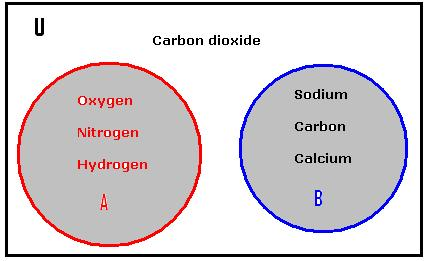In Venn diagram, the universal set is represented by a rectangle and sub sets are represented by circles or ovals.

3.2 Example 3 : Let your class have a strength of 22. Assume that out of these, 11 are members of the cricket team. Also assume that you have a Hockey team of 11 players from these 22 students.It is possible that few may be members of both the team and few may not be members of any team.

The Venn diagram drawn on the right helps us to find the players who are members of both the teams and students who are not members of any team.

 Let U represent the set of students in your class. U = { X1,X2,X3………… X22) Let A be the players representing cricket team. A = { X1,X3, X4,X6, X8,X11,X12, X14,X17,X19,X21) Let B be the players representing hockey team. B = { X2,X3,X6.X9,X10,X13,X14,X15,X18,X19,X20} How do we find players who represent both teams? How do you find players who are not in any team? We find that { X3,X6,X14,X19}  is the set  of  four players who are in both the teams. The set AB ={ X1,X2, X3,X4, X6,X8, X10,X11,X12,X13,X14,X15,X17,X18, X19,X20, X21) is the set of players who are in Cricket team or Hockey team. This is the grey+ yellow+grey colored portion of the figure. We can represent these sets using Venn diagrams as given on the right hand side. The section shaded in yellow color is the set of students who are in both the teams and is represented by AB. We also find that {X5,X7,X9,X16,X22} is the set of  five students who are not in any team.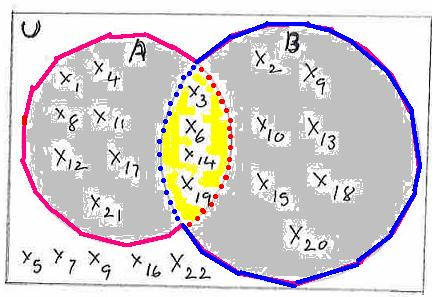3.2 Example 4 : Let A = { 2,4,6,8}, Let B = { 2,4,6}= { }

 Observations: Since in a set, the elements are written only once AA = {2,4,6,8} = A   Sinceis a subset of every set , A={ 2,4,6,8} = A   If BA then BA = {2, 4,6,8}= A   IF BA then AB = {2,4,6} = B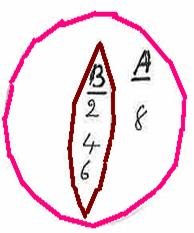3.2 Problem  1: Draw the Venn diagram for ABC and ABC if  A = {1,5,7,9} and B={1,3,7,10} and C= {5,6,7,8,9,10}

Solution :

 Set A is represented by the green colored circle. Set B  is represented by the blue colored circle. Set C is represented by the red colored circle. (AB)C =  ({1,3,5,7,9,10}){5,6,7,8,9,10} = {1,3,5,6,7,8,910} (AB)C  = ( { 1,7}{5,6,7,8,9,10} = {7}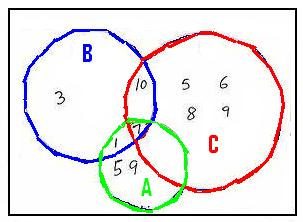3.2 Problem 2: A = {x: x2-8x+12 =0} and B = {x:  x2-6x+8 =0} Find AB and AB

Solution :

 We know that x2-8x+12 = (x-6)(x-2). Thus x2-8x+12 = 0  is true when  x=6 or x=2 We know that x2-6x+8 = (x-4)(x-2). Thus  x2-6x+8  = 0 is true when  x=4 or x=2   Therefore A = {6,2) (represented by blue colored circle) and B= {4,2} (represented by green colored circle). Hence AB = {6, 4, 2}, AB ={2}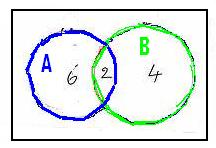Let U = (Black, Pink, Brown, Purple, Violet, Indigo, Blue, Green, Yellow, Orange, Red} A = {Violet, Indigo, Blue, Green, Yellow, Orange, Red}   The sets can be represented by a Venn diagram as shown in the adjoining figure.   Let us consider the set {Black, Pink, Brown, Purple} What is special about this set? This set has elements of U which are not in A. It is called ‘complement' of set A and is denoted by A1. So A1={Black, Pink, Brown, Purple} Definition :  A set is said to be ‘complement’ to another set U, if the elements in that set has elements which are in U but not in itself . The complement of set A is denoted by A1. We notice that A1U and AA1=U and AA1=( The sets A and A1  do not have any common element). (A1)1 = {elements of U which are not in A1} = {Violet, Indigo, Blue, Green, Yellow, Orange, Red}= A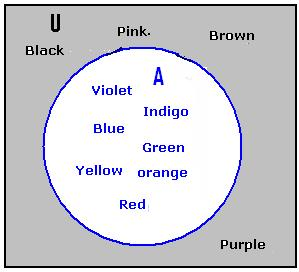3.2 Problem 3:  If U = {Natural numbers less than 9}, A = {Even numbers less than 9}, B = {Prime numbers less than 9}

Find A1B1 and A1B1

Solution :

 We have U = {1,2,3,4,5,6,7,8}, A = {2,4,6,8}, B = {2,3,5,7}   A1= { 1,3,5,7}( covered by blue colored quadrilateral ), B1= { 1,4,6,8}( covered by red colored quadrilateral) A1B1  = {1,3,4,5,6,7,8} A1B1= {1}   Let us find (AB)1 and (AB)1 AB= {2,3,4,5,6,7,8} (AB)1= {1} AB =  {2}( covered by orange colored square) (AB)1= { 1,3,4,5,6,7,8} What do we notice?   (AB)1= A1B1  and (AB)1= A1B1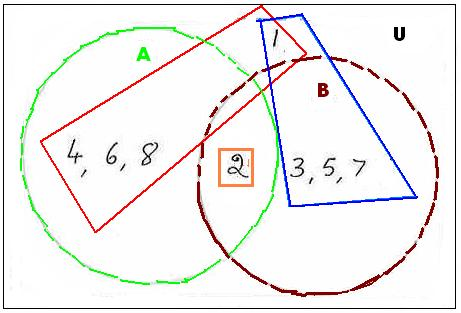If A and B are two sets then the ‘difference’ set (A-B) is defined as a set which has elements of A but not of B.

3.2 Example 5 :  Let H = {Squares of natural numbers less than 36} J = {1, and multiples of 2 less than 34} Find  HJ   H-J and J-H

 We have H = { 1,4,9,16,25}, J = {1,2,4,8,16,32} HJ  = {1,4,16}( covered by orange colored rectangle) H- J = {elements of H not in J} = {9,25}(covered by red colored rectangle) J - H = {elements of J not in H} = {2,8,32}(covered by blue colored rectangle)   Notice that H-J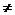J-H   Observations: For any sets U and A ()1=U and(U)1=A-A=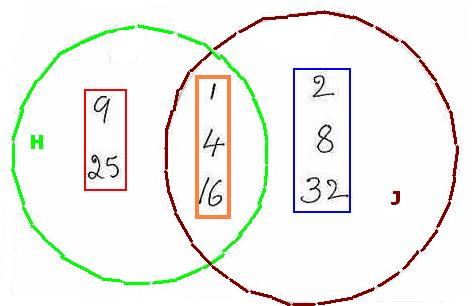3.2 Summary of learning

 No Points to remember 1 Definitions of sets(Union, Intersection, compliment, difference) 2 Venn diagram